#The Wolfram Language:Fast Introduction for Programmers

Show additional notes for:
All sectionsGet Started

# Function DefinitionsView as Video

In the Wolfram Language, function definitions are just assignments that give transformation rules for patterns.

Notes for Java programmers:

Unlike methods in the object-oriented Java language, Wolfram Language functions are not restricted to a class hierarchy.

Define a function of two arguments named x and y:

In:=1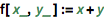`f[x_, y_] := x + y`

Notes for Java programmers:

In basic Wolfram Language function definitions, parameter names are followed by _ ("blank"). Unlike Java methods, Wolfram Language functions will accept any type of data by default.

Notes for Python programmers:

In basic Wolfram Language function definitions, parameter names are followed by _ ("blank"), allowing parameters to correspond to arbitrary symbolic patterns. This enables powerful and flexible function definitions not possible by default in Python.

Use the definition:

In:=2`f[4, a]`
Out=2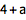Clear the definition:

In:=3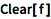`Clear[f]`

Functions can be defined by specifying their values in a sequence of cases:

In:=1`f = 2`
Out=1In:=2`f = 3`
Out=2Any undefined case is left untransformed:

In:=3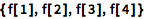`{f, f, f, f}`
Out=3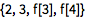You can use /; to restrict a definition to apply only when a particular condition holds:

In:=4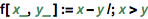`f[x_, y_] := x - y /; x > y`

Notes for Java programmers:

Using /; in the Wolfram Language is like a shortened If statement.

Notes for Python programmers:

Using /; in the Wolfram Language is like an if statement that can be applied to a function, allowing a function to flexibly choose a definition based on a condition.

You can define transformations for any left-hand side—a convenient way to "destructure" arguments:

In:=1`f[{x_, y_}, z_] := {x + z, y - z}`

In:=2`f[{5, 6}, q]`
Out=2The Wolfram Language doesn't have types as such; everything is a symbolic expression. The "head" of an expression can be used as a kind of generalized type designator.

Give different definitions for what f should do when applied to a "u object" or a "v object":

In:=1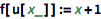`f[u[x_]] := x + 1`

In:=2`f[v[x_]] := x - 1`

Notes for Java programmers:

This is similar to overloading a Java method for different data types, except that Wolfram Language functions can be overloaded more generally, based on the symbolic structure of their arguments.

Notes for Python programmers:

A Wolfram Language function can use built-in pattern matching to change its behavior based on the symbolic structure of any of its arguments. Achieving this in Python would require fairly elaborate custom code or third-party libraries.

QUICK REFERENCE: Defining Variables and Functions

Which of the following defines a function f that adds two numbers (e.g., f[3, 4] == 7)?

Which definition would make {f, f, f, f} evaluate to {f, 5, 6, f}?

If the function f is defined as f[x_, y_] := x + y, then which of these is the value of f[a, b]?